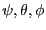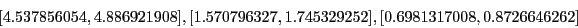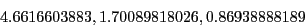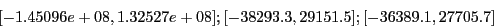Next: Stopping the general solving Up: Examples Previous: Example 3   Contents

Example 4

In this example (see section 15.1.3) we deal with a complex problem of three equations in three unknowns. We are looking for a solution in the domain:The system has a solution which is approximatively:This problem is extremely ill conditioned as for the TestDomain the equations intervals are:The name of the test program is Test_Solve_JH_General.

If we use epsilonf=0.1 and epsilon=0, we get the first solution with the following number of boxes and computation time:

 full bisection smear bisection direct storage 71, 31200ms 38, 20220ms reverse storage 35, 14710ms 38, 20280ms
We need 71 boxes in the full bisection mode to determine that this solution is unique and 38 boxes in the smear bisection mode.

Note that we may improve the efficiency of the procedure by using simplification procedures such as the 2B (section 2.17) and the 3B method. An interesting point in this example is that the bisection mode 1 (bisecting along the variable whose interval has the largest diameter) is more effective than using the bisection mode 2 (using the smear function) with 33 boxes against 38 for the mode 2 for epsilonf=1e-6 and epsilon=1e-6. This can easily been explained by the complexity of the Jacobian matrix elements that leads to a large overestimation of their values when using interval: in that case the smear function is not very efficient to determine which variable has the most influence on the equations. But it must be noted that the use of the Hessian allows to reduce this overestimation and consequently the differences in term of used boxes between the two bisection mode is slightly reduced compared to the one we have observed when using only the Jacobian (see section 2.4.3.4).Next: Stopping the general solving Up: Examples Previous: Example 3   Contents
Jean-Pierre Merlet 2012-12-20